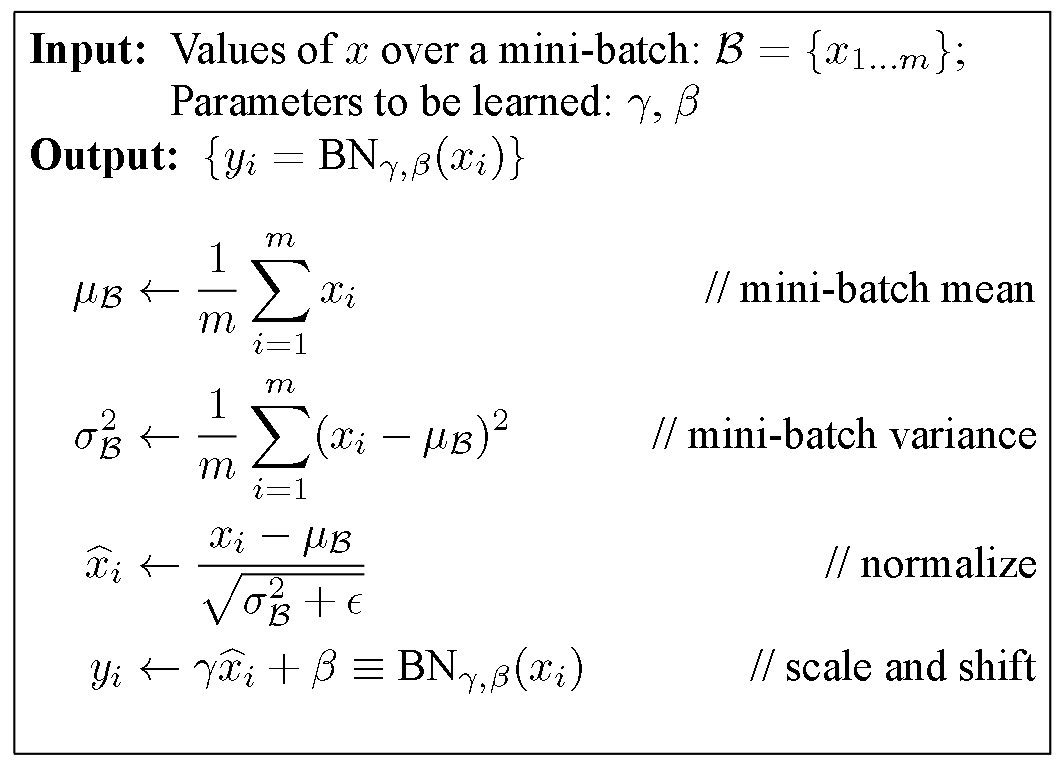# Data Analysis

## Neural Networks 11

1. Some materials are taken from machine learning course of Victor Kitov

# Let's recall previous lecture¶

## Boosting, Ensembles¶

• Construction of multiple models to increase model quality
• In parallel (Bagging, Blending, Stacking, Random Forest)
• Sequentially (Boosting)
• Works great!
• Hard to interpret

# Neural Networks¶

## History¶

• Neural networks originally appeared as an attempt to model human brain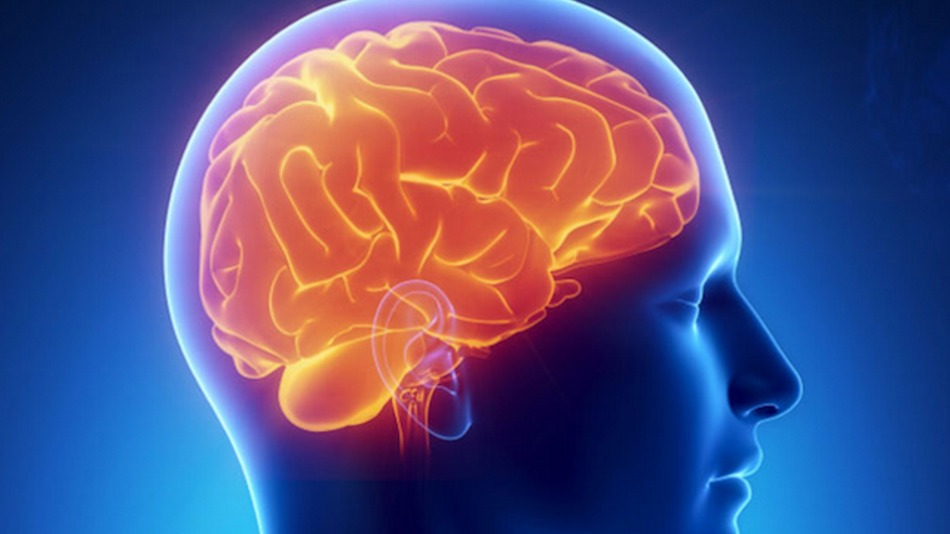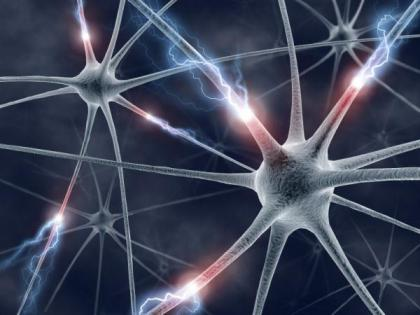• Human brain consists of multiple interconnected neuron cells

• cerebral cortex (the largest part) is estimated to contain 15-33 billion neurons
• communication is performed by sending electrical and electro-chemical signals
• signals are transmitted through axons - long thin parts of neurons.

## History¶

• 1943 – The first mathematical model of a neural network (Walter Pitts and Warren McCulloch)
• 1957 – Setting the foundation for deep neural networks (Frank Rosenblatt)
• 1965 – The first working deep learning networks
• 1979-80 – An ANN learns how to recognize visual patterns
• 1982 – The creation of the Hopfield Networks
• 1989 – Machines read handwritten digits (Yann LeCun)
• 1997 – Long short-term memory was proposed (Jürgen Schmidhuber and Sepp Hochreiter)
• 1998 – Gradient-based learning (Yann LeCun)
• 2011 – Creation of AlexNet
• 2014 – Generative Adversarial Networks (GAN)

## Simple model of a neuron¶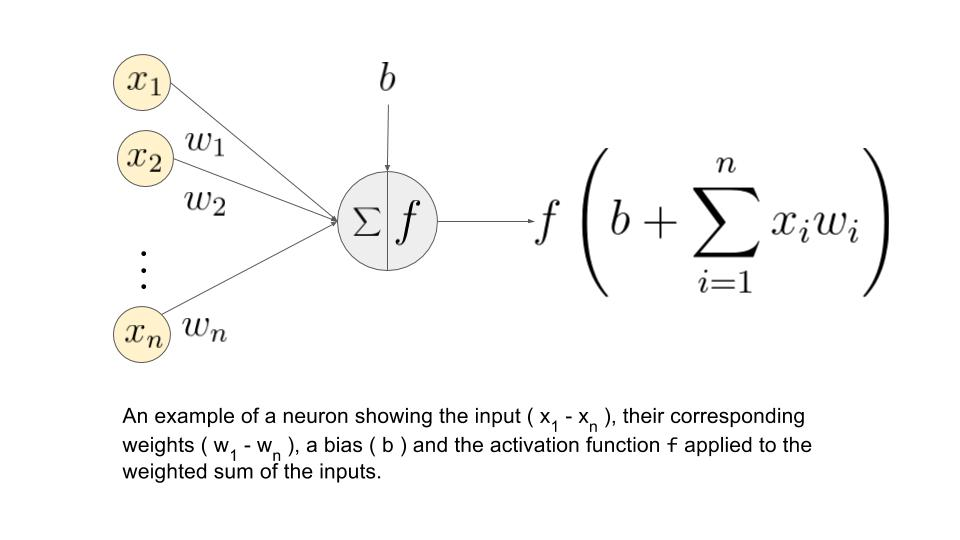• Neuron get's activated in the half-space, defined by $b+w_{1}x^{1}+w_{2}x^{2}+...+w_{D}x^{D}\ge0$.
• Each node is called a neuron
• Each edge is associated a weight
• Constant feature $b$ stands for bias (some times reffered as $w_0$)

## Multilayer perceptron architecture¶

• Hierarchically nested set of neurons.
• Each node has its own weights.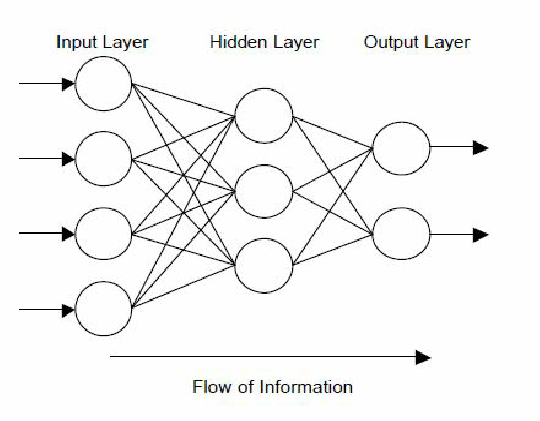This is structure of multilayer perceptron - acyclic directed graph.

## Layers¶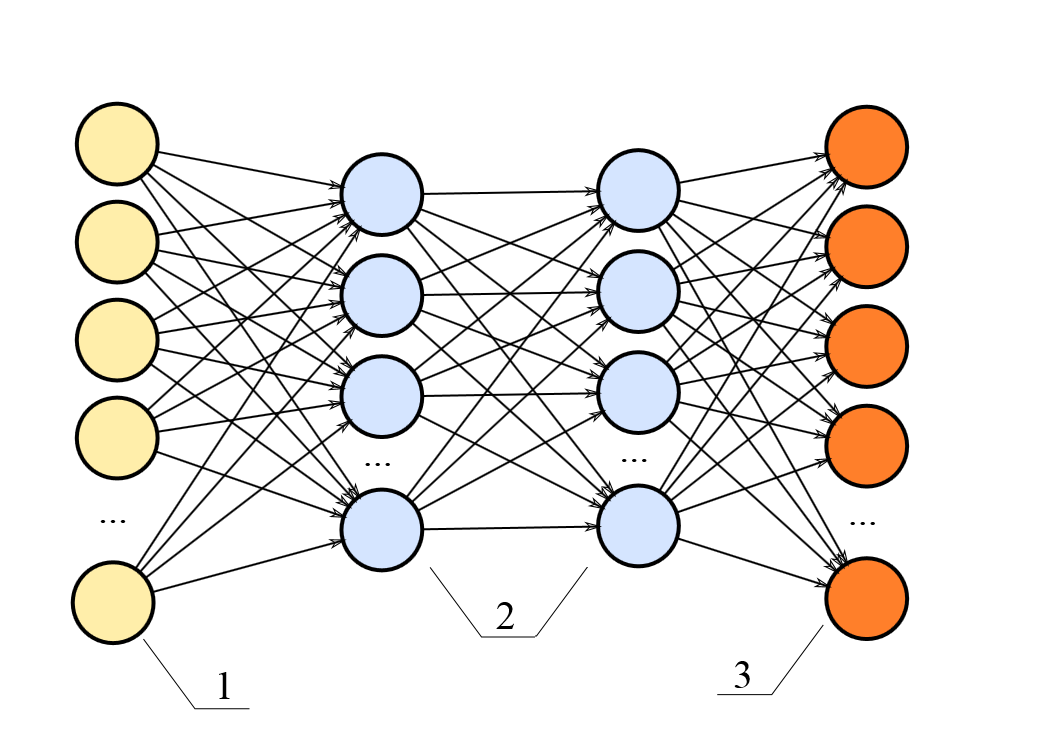• Structure of neural network:
• 1-input layer
• 2-hidden layers
• 3-output layer

## Continious activations¶

• Pitfall of $\mathbb{I}[]$: it causes stepwise constant outputs, weight optimization methods become inapliccable.
• We can replace $\mathbb{I}[w^{T}x+w_{0}\ge0]$ with smooth activation $f(w^{T}x+w_{0})$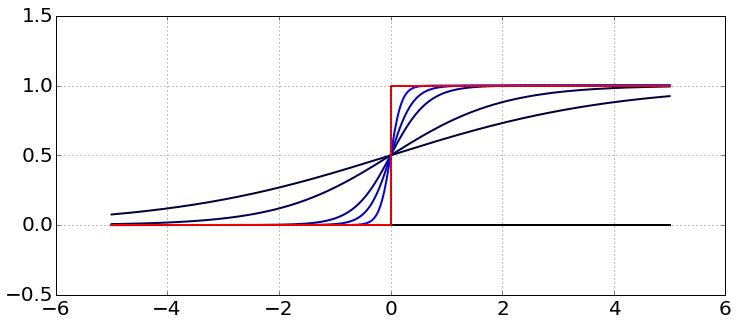## Typical activation functions¶

• sigmoidal: $\sigma(x)=\frac{1}{1+e^{-x}}$
• 1-layer neural network with sigmoidal activation is equivalent to logistic regression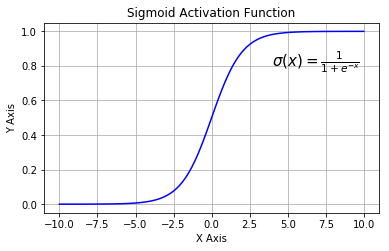• hyperbolic tangent: $tangh(x)=\frac{e^{x}-e^{-x}}{e^{x}+e^{-x}}$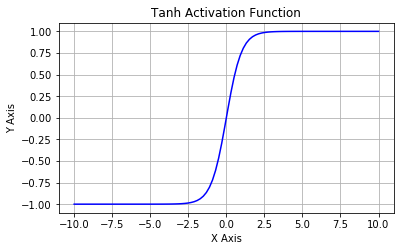## Typical activation functions¶

• ReLU: $f(x)=[x]_{+}$.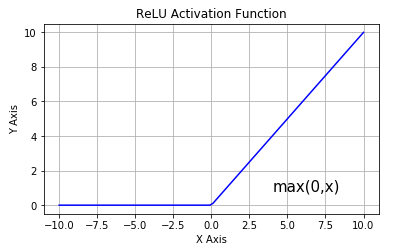## Activation function zoo¶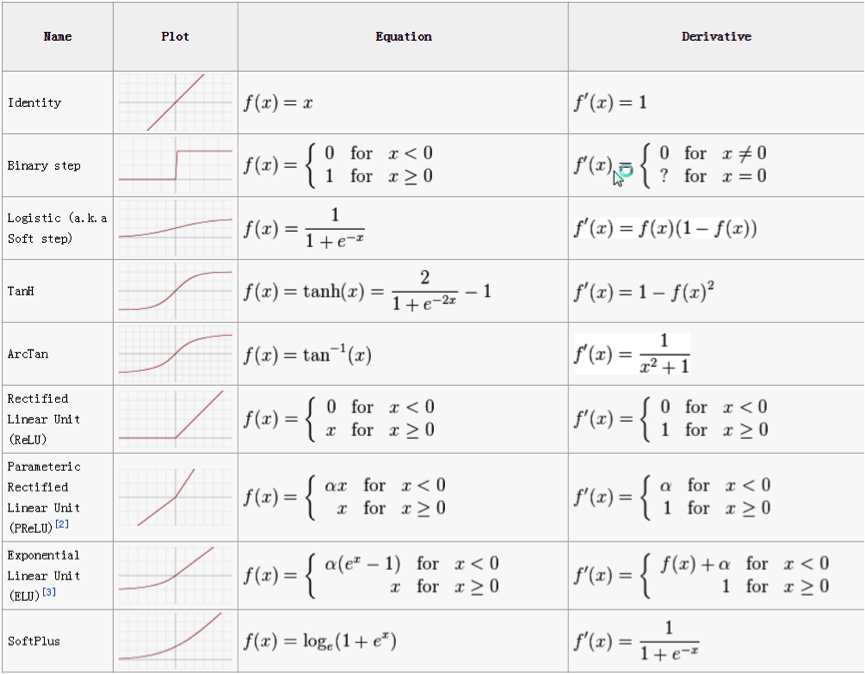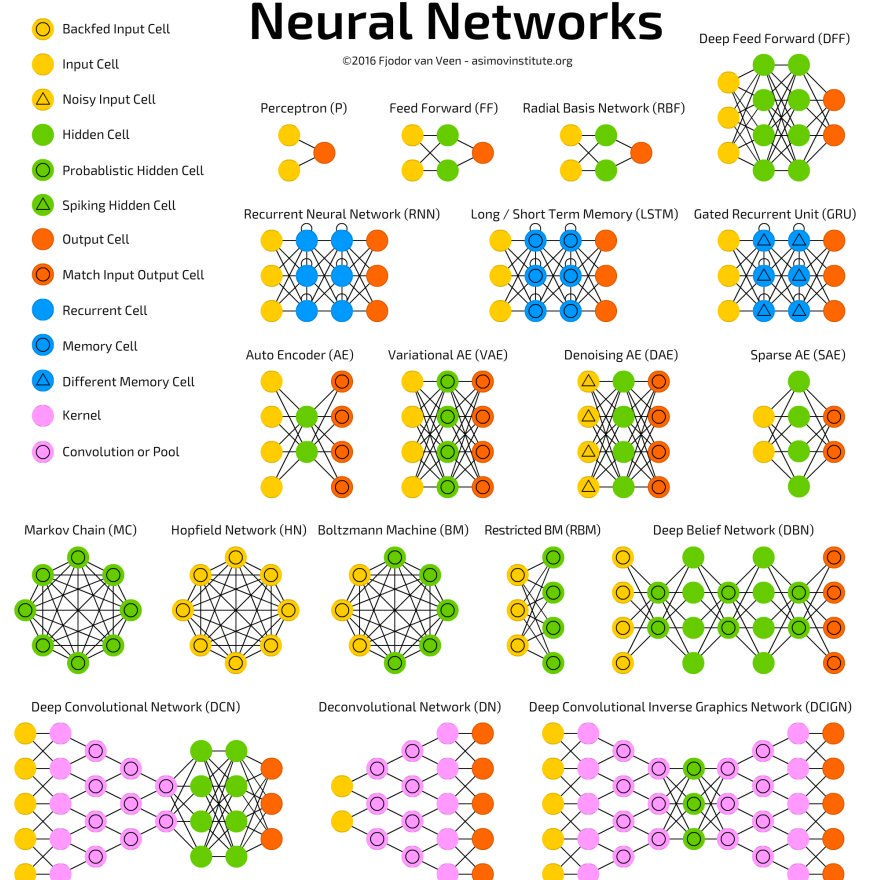# Output Generation¶

## Output generation¶

• Forward propagation is a process of successive calculations of neuron outputs for given features.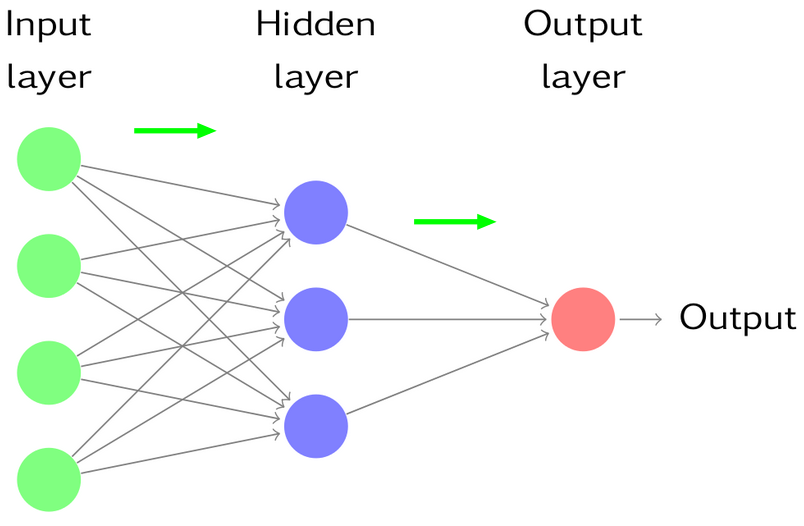## Definition details¶

• Label each neuron with integer $j$.
• Denote: $I_{j}$ - input to neuron $j$, $O_{j}$ - output of neuron $j$
• Input to neuron $j$: $I_{j}=\sum_{k\in inc(j)}w_{kj}O_{k}+w_{0j}$,
• Output of neuron $j$: $O_{j}=f(I_{j})$.

• $w_{0j}$ is the bias term
• $f(x)$ is the activation function
• $inc(j)$ is a set of neurons with outgoing edges incoming to neuron $j$.
• further we will assume that at each layer there is a vertex with constant output $O_{const}\equiv1$, so we can simplify notation
$$I_{j}=\sum_{k\in inc(j)}w_{kj}O_{k}$$

## Activations at output layer¶

• Regression: $f(I)=I$ (linear activation)
• Classification:
• binary: $y\in\{+1,-1\}$ $$f(I)=p(y=+1|x)=\frac{1}{1+e^{-I}}$$
• multiclass: $y\in{1,2,...C}$ $$f(I_{1},...I_{C})=p(y=j|x)=\frac{e^{I_{j}}}{\sum_{k=1}^{C}e^{I_{k}}},\,j=1,2,...C$$ where $I_{1},...I_{C}$ are inputs of output layer.

## Generalizations¶

• each neuron $j$ may have custom non-linear transformation $f_{j}$
• weights may be constrained:
• non-negative
• equal weights
• etc.
• layer skips are possible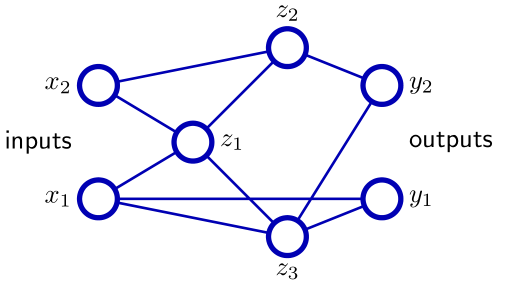• Not considered here: RBF-networks, recurrent networks.

## Number of layers selection¶

• Number of layers usually denotes all layers except input layer (hidden layers+output layer)

• Classification:

• single layer network selects arbitrary half-spaces
• 2-layer network selects arbitrary convex polyhedron (by intersection of 1-layer outputs)
• therefore it can approximate arbitrary convex sets
• 3-layer network selects (by union of 2-layer outputs) arbitrary finite sets of polyhedra
• therefore it can approximate almost all sets with well defined volume

## Number of layers selection¶

• Regression:
• single layer can approximate arbitrary linear function
• 2-layer network can model indicator function of arbitrary convex polyhedron
• 3-layer network can uniformly approximate arbitrary continuous function (as sum weighted sum of indicators convex polyhedra)
• Sufficient amount of layers
Any continuous function on a compact space can be uniformly approximated by 2-layer neural network with linear output and wide range of activation functions (excluding polynomial).
• In practice often it is more convenient to use more layers with less total amount of neurons
• model becomes more interpretable and easy to fit.

# Neural network optimization¶

## Network optimization: regression¶

• Single output:
$$\frac{1}{N}\sum_{n=1}^{N}(\widehat{y}_{n}(x_{n})-y_{n})^{2}\to\min_{w}$$
• K outputs
$$\frac{1}{NK}\sum_{n=1}^{N}\sum_{k=1}^{K}(\widehat{y}_{nk}(x_{n})-y_{nk})^{2}\to\min_{w}$$

## Network optimization: classification¶

• Two classes ($y\in\{0,1\}$):
$$\prod_{n=1}^{N}p(y_{n}=1|x_{n})^{y_{n}}(1-p(y_{n}=1|x_{n})){}^{1-y_{n}}\to\max_{w}$$
• $C$ classes ($y_{nc}=\mathbb{I}\{y_{n}=c\}$):
$$\prod_{n=1}^{N}\prod_{c=1}^{C}p(y_{n}=c|x_{n})^{y_{nc}}\to\max_{w}$$
• In practice log-likelihood (cross-entropy) is maximized

## Neural network optimization¶

• Let $L(\widehat{y},y)$ denote the loss function of output
• We may optimize neural network using gradient descent:

  k=0
initialize randomly w_0 # small values for sigmoid and tangh

while stop criteria not met:
w_k+1 := w_k - alpha * grad(L(w_k))
k := k+1
• Standardization of features makes gradient descend converge faster

• But how exactly do we efficiently calculate grad(L(w_k))?

# Backpropagation algorithm¶## Idea¶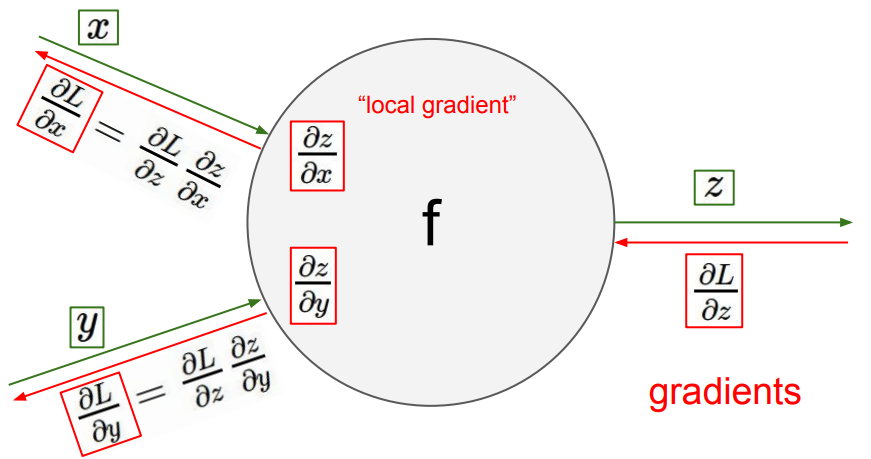## Definitions¶

• Denote $w_{ij}$ as weight of edge, connecting $i$-th and $j$-th neuron
• Define $\delta_j = \frac{\partial L}{\partial I_j} = \frac{\partial L}{\partial O_j}\frac{\partial O_j}{\partial I_j}$
• Since $L$ depends on $w_{ij}$ through the following functional relationship $L(w_{ij}) = L\left(O_j\left(I_j(w_{ij})\right)\right)$, using the chain rule we get: $$\frac{\partial L}{\partial w_{ij}} = \frac{\partial L}{\partial I_j}\frac{\partial I_j}{\partial w_{ij}} = \delta_j O_i$$ because $\frac{\partial I_j}{\partial w_{ij}} = \frac{\partial}{\partial w_{ij}} \left(\sum\limits_{k\in inc(j)} w_{kj} O_k\right) = O_i$, where $inc(j)$ is a set of neurons with outgoing edges to neuron $j$

## Output layer¶

• If neuron $j$ belongs to the output node, then error $\frac{\partial L}{\partial O_j}$ is calculated easily
• For output layer $\delta_j$ are calculated directly: $$\delta_j= \frac{\partial L}{\partial O_j}\frac{\partial O_j}{\partial I_j} = \frac{\partial L}{\partial O_j} f'(I_j) \qquad (1)$$
• Example (single point $x$ and true vector of outputs $(y_1,\dots,y_{|OL|})$:
• For $L = \frac{1}{2}\sum\limits_{j\in OL}(O_j - y_j)^2$ and sigmoid activation $$\frac{\partial L}{\partial O_j} = O_j - y_j$$
• Sigmoid activation function $O_j = \sigma(I_j)$: $$f'(I_j) = \sigma(I_j)(1-\sigma(I_j)) = O_j(1-O_j)$$
• finally $$\delta_j = (O_j - y_j)O_j(1-O_j)$$

## Inner layer¶

• If neuron $j$ belongs to some hidden layer, denote $out(j) = \{k_1, k_2, \dots, k_m\}$ the set of all neurons, receiving output of neuron $j$ as their input
• The effect of $O_j$ on $L$ is fully absorbed by $I_{k_1},I_{k_2},\dots,I_{k_m}$, so $$\frac{\partial L(O_j)}{\partial O_j} = \frac{\partial L(I_{k_1},I_{k_2},\dots,I_{k_m})}{\partial O_j} = \sum\limits_{k\in out(j)} \left( \frac{\partial L}{\partial I_k} \frac{\partial I_k}{\partial O_j} \right) = \sum\limits_{k\in out(j)} \left(\delta_k w_{jk}\right)$$
• So for layers other than output layer we have: $$\delta_j = \frac{\partial L}{\partial I_j} = \frac{\partial L}{\partial O_j}\frac{\partial O_j}{\partial I_j} = \sum\limits_{k\in out(j)} \left(\delta_k w_{jk}\right) f'(I_j) \qquad (2)$$
• Weight derivatives are calculated using errors and outputs: $$\frac{\partial L}{\partial w_{ij}} = \frac{\partial L}{\partial I_j}\frac{\partial I_j}{\partial w_{ij}} = \delta_jO_i \qquad (3)$$

## Backprop¶

1. Forward propagate $x_n$ to the neural network, store all inputs $I_j$ and outputs $O_j$ for each neuron
2. Calculate $\delta_i$ for all $i \in$ output layer using $(1)$ $$\delta_j = \frac{\partial L}{\partial O_j} f'(I_j)$$
3. Propagate $\delta_i$ from final layer back layer by layer $(2)$ $$\delta_j = \sum\limits_{k\in out(j)} \left(\delta_k w_{jk}\right) f'(I_j)$$
4. Using calculated deltas and outputs calculate $\frac{\partial L}{\partial w_{ij}}$ with $(3)$ $$\frac{\partial L}{\partial w_{ij}} = \delta_jO_i$$ And update weights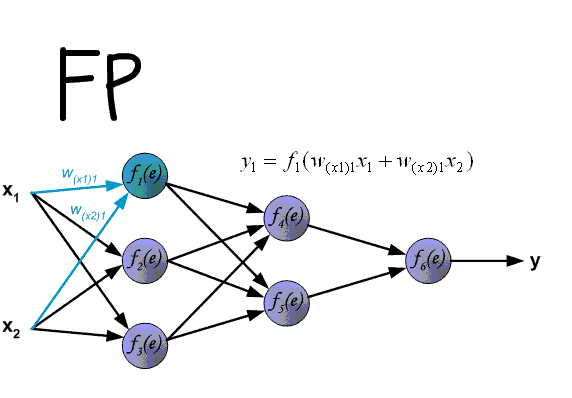### Multiple local optima problem¶

• Optimization problem for neural nets is non-convex.
• Different optima will correspond to:

• different starting parameter values
• different training samples
• So we may solve task many times for different conditions and then

• select best model
• alternatively: average different obtained models to get ensemble
• And/Or use some complex optimization methods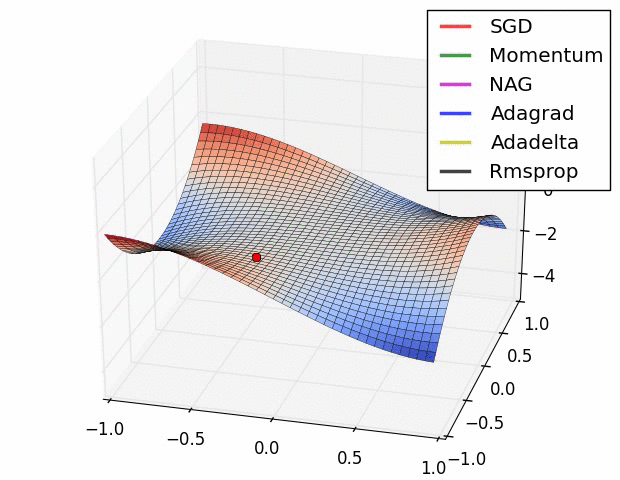In :
x = np.linspace(-10, 10, 1000)
gr_sigm = sigmoid(x)*(1-sigmoid(x))

plt.plot(x, gr_sigm)

Out:
[<matplotlib.lines.Line2D at 0x120210da0>]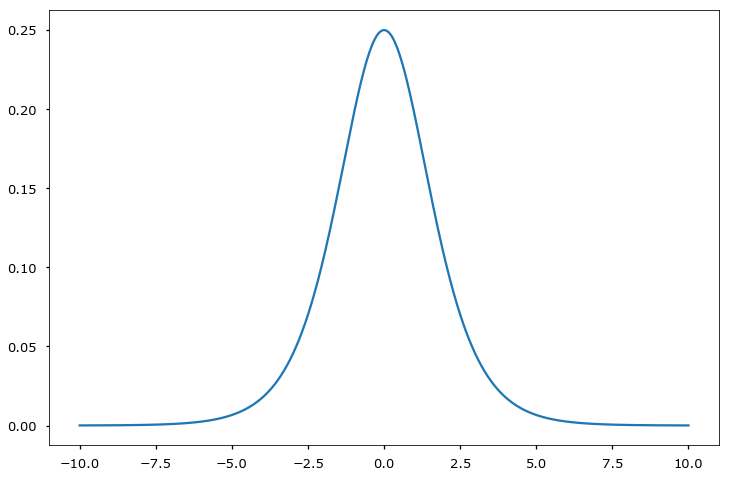• Feature scaling
• Careful weight initialization
• Using ReLU activation function

## Model complexity and overfitting¶

• Constrain model directly:
• constrain number of neurons
• constrain number of layes
• impose constraints on weights
• Take a flexible model
• early stopping (with validation set)
• L2 regularization $$L(w) + \lambda\sum_i w_i^2$$
• Augmentation (more used in convnets)

# Other things to know¶

## Weight Initialization¶

• Poor weight initialization can lead to vanishing or exploding gradient problem
• Variance of a layer affects variance of the next one
• Initialize weights in such manner to keep variance constant (Xavier initialization)
• More here

## Dropout technique¶

• Have L1 and L2 regularization for weights
• Can complement it with Dropout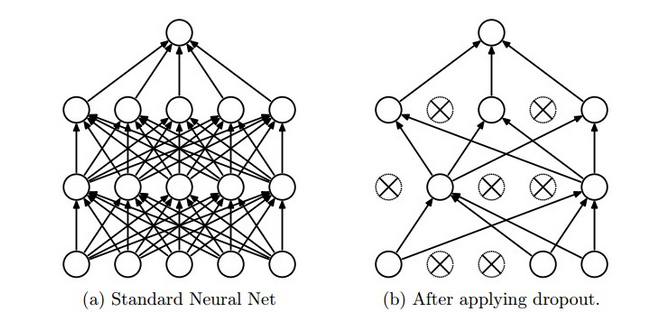• Training: Dropout can be interpreted as sampling a Neural Network within the full Neural Network
• Testing: not applied

## Batch Normalization¶

• Normalize data right before non-linearities (or after ?!)